### Quantum Computing

Welcome to Quantum Computing! Ours is an endeavor that's been promised to upend everything from codebreaking, to drug development, to machine learning. With so much hype, it's easy to get lost marveling at the possibilities, without grasping what quantum computing actually is.

Our focus is learning how to exploit the laws of quantum mechanics in order to compute. For the most part, we'll be concerned with the structures of quantum mechanics that are useful to that end — and remain blissfully ignorant about detailed quantum physics.

By the end, this course will give you a wide-eyed take on the algorithms and technology that underlie what you read in news reports about quantum computing. But before that, we need to see the big picture, which we can do by answering two questions:

1. what is the nature of quantum computing, and
2. why do scientists think it will lead to speedups on a variety of important problems?

# The Nature of Computation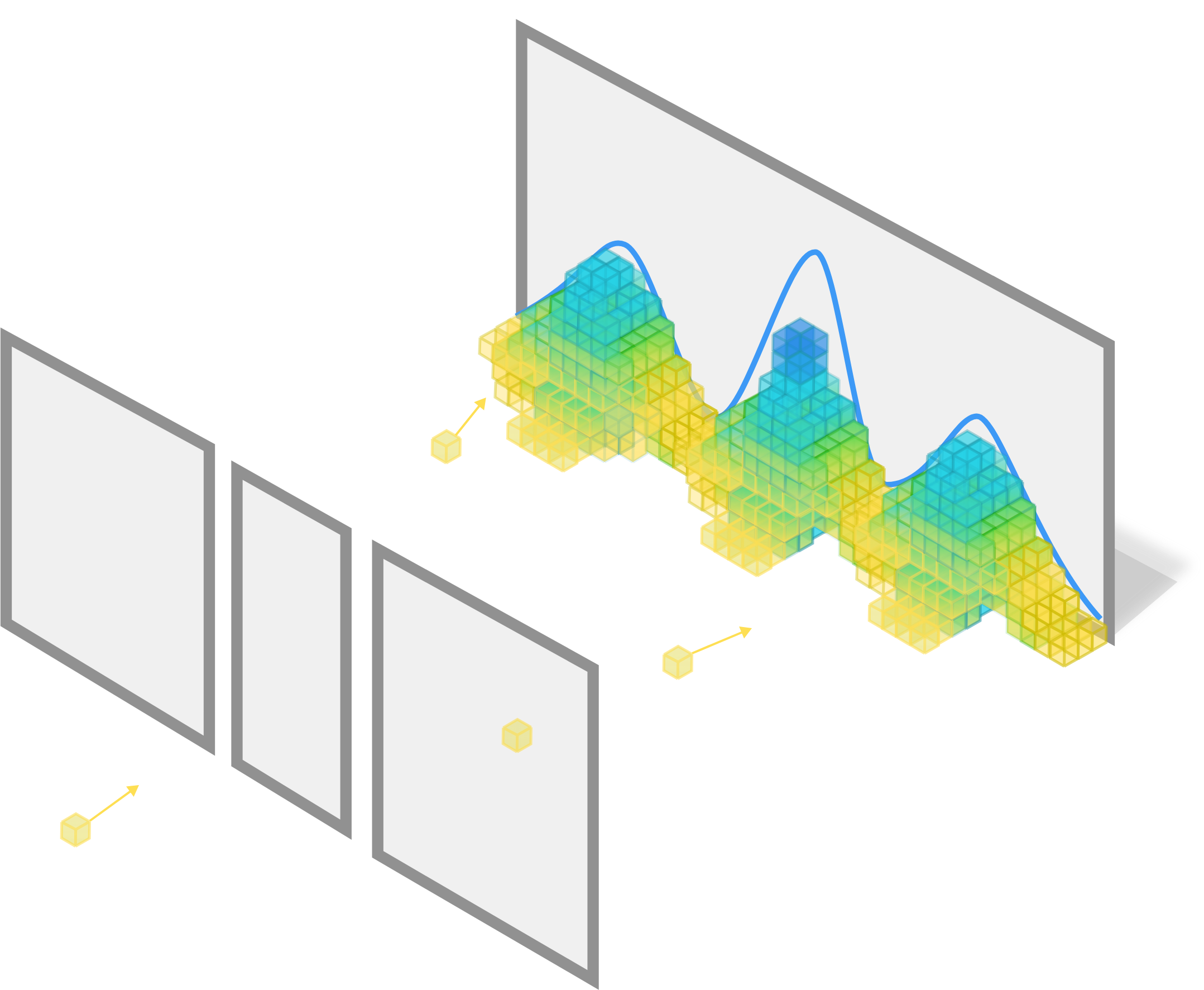Individual photons passing through a double slit could be detected anywhere, but will cumulatively form a probability distribution.

In the dawn of the $20^\text{th}$ century, deterministic predictions from classical mechanics were failing to explain new experiments.

A new theory was necessary to describe the results of experiments performed with quantum objects; photons, electrons, and other microscopic particles. Quantum mechanics forgoes deterministic predictions, and instead embraces the concept of probability. In other words, quantum mechanics can predict the statistical distribution of the outcome of many experiments, though any single experiment is a random event.

As quantum computing is a story of the subordination of quantum objects, it's fitting to start our exploration with a simple game of our own. We'll play it with photons.

# The Nature of Computation

This simple game of chance is well-known the world over. In Japan it's called pachinko, and on American game shows it's known as Plinko. To mathematicians and physicists, it's sometimes called a Galton board.

Whatever we call it, this game is a simple demonstration of how probabilities can emerge from a mechanical object. Each bin at the bottom is associated with a different prize, and the thrill is to watch as your fate is determined by the random bounces of the ball off the pegs.

In this quiz, we'll remake the game with quantum objects: a photon instead of a ball, and beam splitters at every corner.

At every bounce, the ball and the photon behave similarly: both have their choice of two paths: either to the right, or to the left, each with equal probability.

What is the most likely outcome of these games?

# The Nature of Computation

With a single photon, the quantum game behaves much like the mechanical version with the bouncing ball; the more possible paths that lead to a given outcome, the more likely it is.

But if we shoot two photons in the top of the grid, things get more interesting. When two or more photons meet somewhere on the grid, they can interfere with one another. Interference can lead to anything from the two photons adding constructively and appearing like two photons, to cancelling each other out entirely as if there were no photons at all.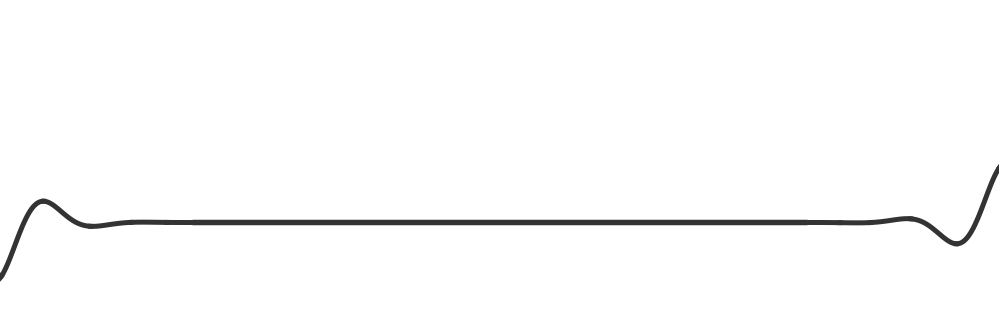Thanks to this interference, we don't have any better way to find where the photons will most likely end up than to trace out all the paths each photon can take and calculate the interference effects where they meet.

As deep as this is (very deep), we're not concerned here with the physics mystery, we're concerned with the last point:

To predict the end state of a quantum object, we need to account for all the paths it could have taken to get there.

# The Nature of Computation

How difficult is it to computationally predict the outcomes of this quantum game?

If we shoot two photons into the top, each will follow a probabilistic path through the grid. Depending on the photon source and the path they take, two photons arriving at the same point may combine to appear like two photons, or cancel each other out completely.

Simulating this game on a computer is a massive undertaking: the only way we know of to calculate the probability of finding a photon at each counter is to trace out every possible combination of paths for each photon and calculate the interference effects where the photons meet (indicated here in red).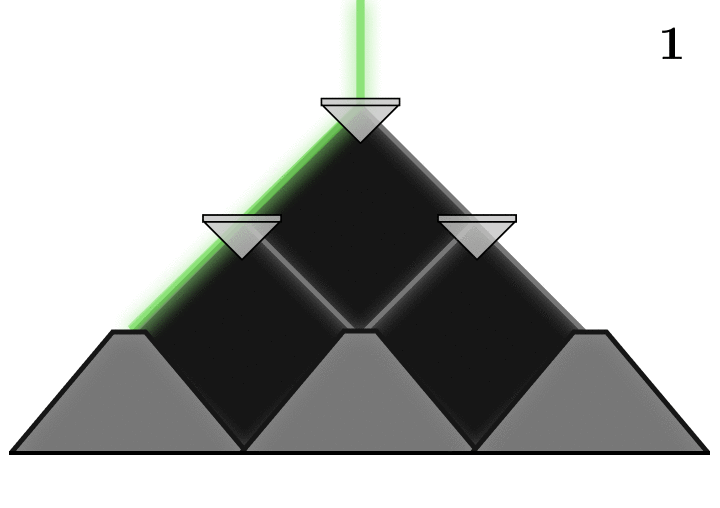In this simple case with two rows of beam splitters and two photons, each photon can take one of four paths, leading to sixteen different combinations of paths for interference calculations. As the game gets larger, more and more paths will end up meeting and interfering.

# The Nature of Computation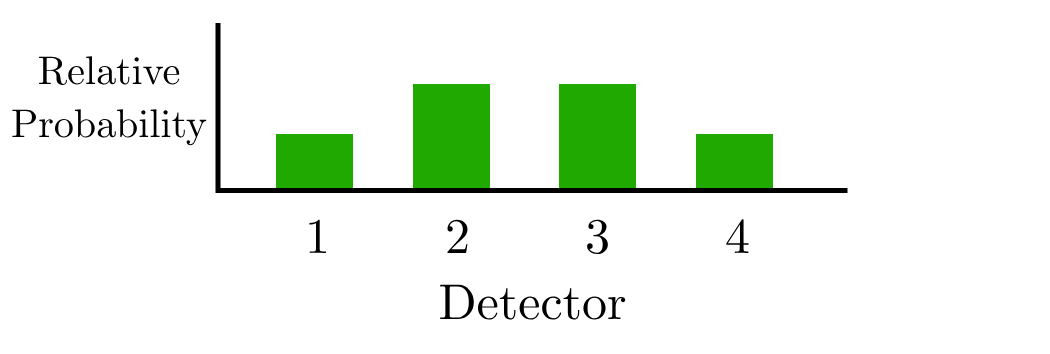For each of the path combinations, calculating the interference between them is quick and easy, and takes $t_c$ seconds.

How much time does it take to calculate the photon distribution for a $3$-layer quantum game with two photons?

Hint: Even if the photons don't meet in some of the combinations, we can't know that until we do the calculation; when simulating in this way, this step needs to be repeated for every possible combination.

# The Nature of Computation

Simulating this simple game seems to take a lot of calculations. What if we just build it?

With a beam splitter grid in hand, we can shoot two photons into the top and use photon counters to find the distribution at the bottom experimentally.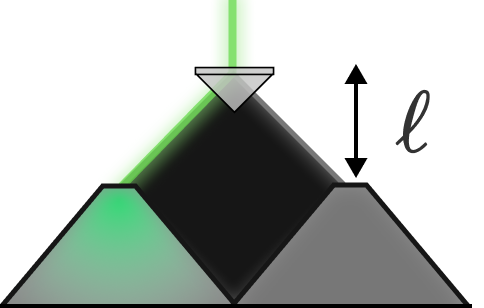With only a pair of photons per experiment, obtaining a reliable estimate for the distribution at the detector involves sampling and averaging millions of photons. For each measurement, the sampling time would be equal to the time the photons take to traverse the gap $\ell$ from the first beam splitter to the row of photon counters at the speed of light. For a single beam splitter with two detectors, this photon sampling takes time $t_q$.

How much time does it take for a 3-layer experimental solve of this quantum game?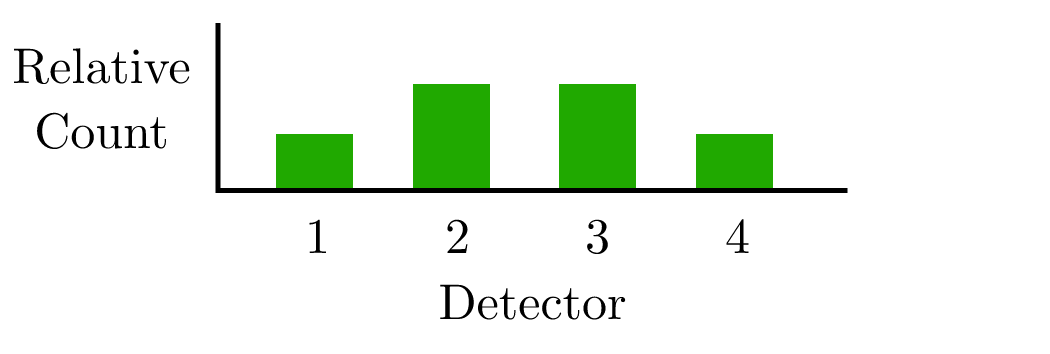Note: In this calculation, we can ignore the distance between the photon source and the first beam splitter since it can be located much closer than the spacing between the layers in an ideal experiment.

# The Nature of Computation

With each layer of beamsplitters we add to the game, the number of paths to consider in our computational simulation increases by a factor of $2$. In general, an $n$-layer game has $2^n$ different paths that each photon can follow.

But to calculate the interference, we consider every possible combination of these $2^n$ paths for two photons. This approach requires calculating interference for $\left( 2^{n} \right) ^2 = 2^{2n}$ paths, which would take at least $2^{2n} \times t_c$ time.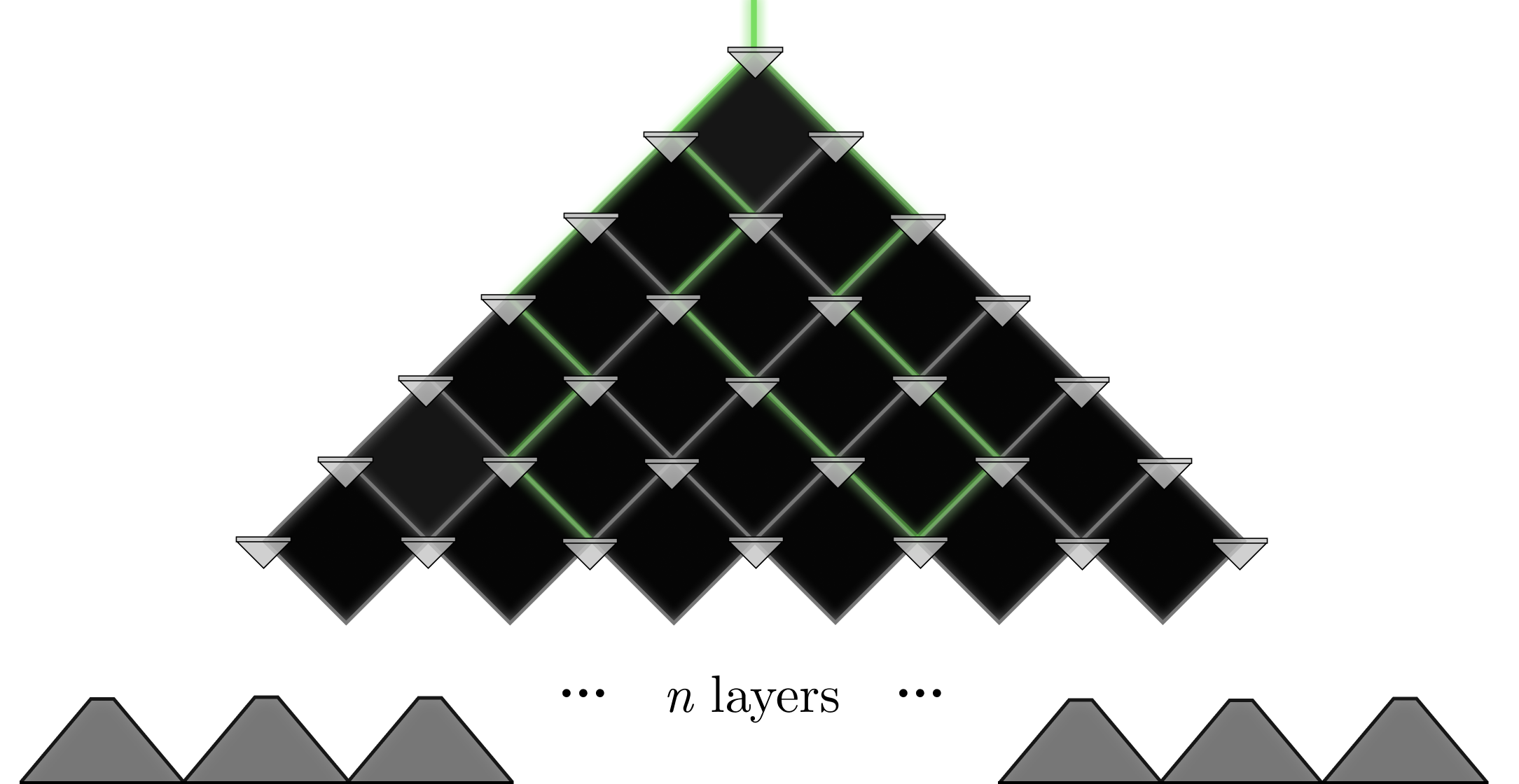How long will a photon counting measurement in an $n$-layer game take experimentally?

# The Nature of Computation

The time required by each of our methods for computing the interference pattern increases at different rates as the number of layers $n$ increases. For a single layer, the time it takes a computer to calculate the likelihood of two paths is likely tiny—much less than a second, while averaging millions of single photon measurements might take several seconds.

But as $n$ increases, the time required for the classical computation $T_\textrm{classic} = 2^{2n} t_c$ increases exponentially with $n$ as the number of possible paths explodes. However, the time required for the quantum experiment $T_q = n t_q$ increases only linearly in $n.$ This is because the quantum objects in the experiment have no obligation to compute paths according to the rules of quantum mechanics, they obey quantum mechanics as a matter of course.

To see how significant this speedup is, we can generously assume $t_q$ to be approximately $\SI{10}{\second}$ (with all the repeat measurements) and $t_c$ to be $\SI{100}{\micro\second}$.

How many layers of beam splitters will it take before our classical computation loses out to the experiment?

# The Nature of Computation

We've been explicit that our pathfinding calculation is the kind of thing we can compute on a classical computer. On the other hand, we've been treating the beam splitter approach more like a laboratory experiment, not a computation. But both methods produce the same information, so if one is a computation, so is the other.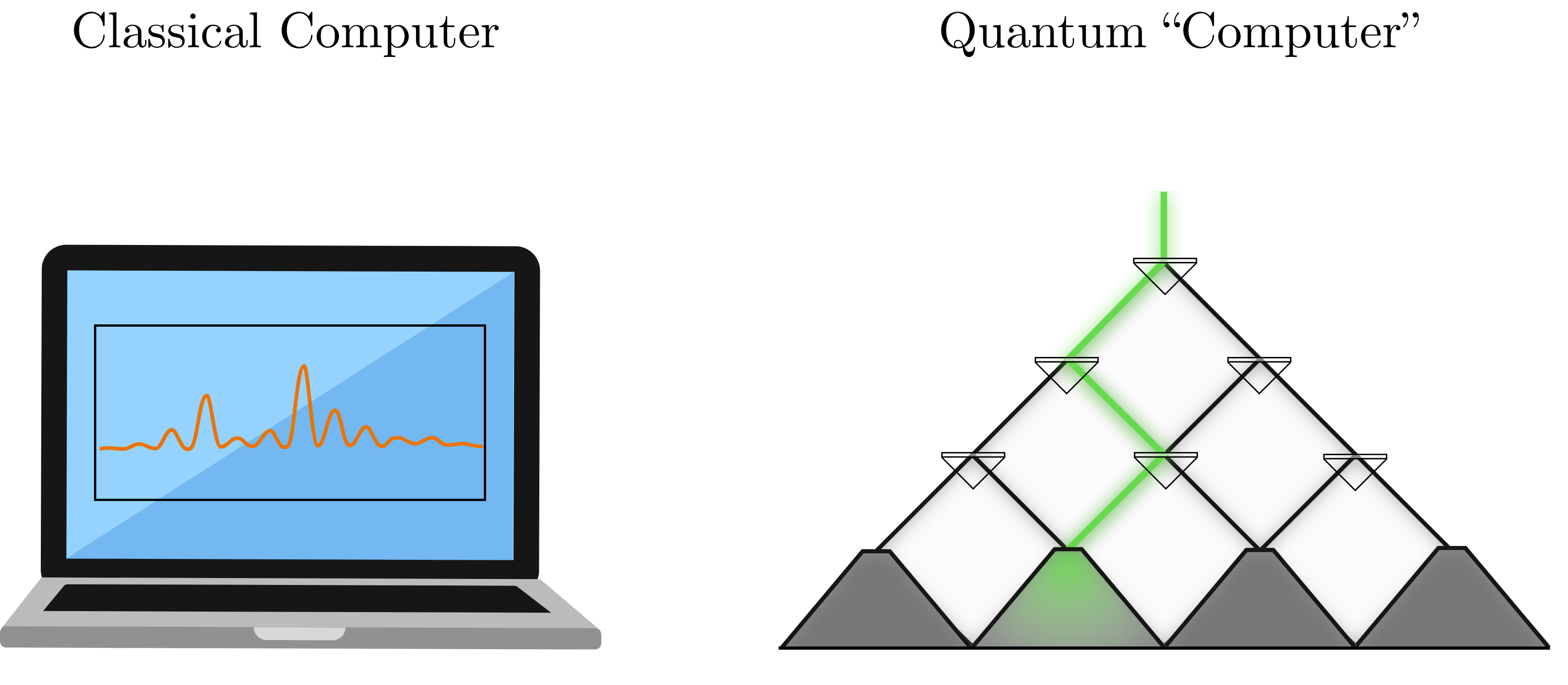The difference is that the experiment gets the result much faster as $n$ increases. In this way, we can see that finding the interference pattern for $n$ layers is a problem that is hard to compute classically, because the resources that it requires grow exponentially as $n$ increases, but is quite easy to compute "quantumly" with an experiment.

We used quotation marks, but this is the basic truth of what's happening in quantum computing—directly employing quantum objects to perform a specific computation. To whatever extent we can map and mold our computational problems onto quantum objects, we'll be able to use it to our advantage. If a problem has an inherent quantum aspect to it, like finding the interference pattern, then we expect a speedup.

And that is the question: "Which of our classical computational problems have a quantum aspect, and how do we see it when it's there?"

# The Nature of Computation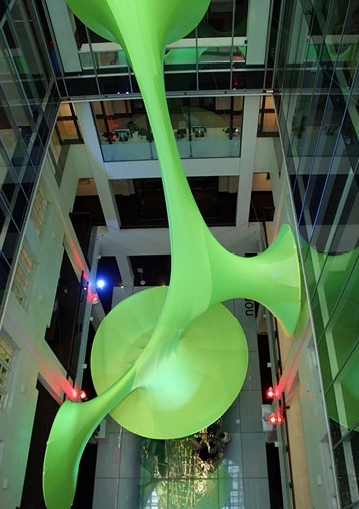Green Void, LAVA (LABORATORY FOR VISIONARY ARCHITECTURE) www.l-a-v-a.net

To get a sense for what it means to map problems onto physical systems, we can consider the computational importance of more familiar physics.

Consider this sculpture, wherein the green fabric makes contact with all the "boundaries" formed by the metal circles. In architecture, as in the study of black holes, a fundamental problem is finding shapes that use the least amount of material to drape between constraints. In fact, the shape we see below is ideal in this way: it is impossible to find any other such smooth shape that contacts the metal rings while using less material.

Finding this surface through computation is not straightforward, and requires algorithms based on sophisticated mathematics. Is there an easier way?

Which phenomenon might be able to "compute" this surface in real time, using the laws of physics?

# The Nature of Computation

Natural systems like soap bubbles have a tendency to wiggle around until they minimize their energy. Set them up, give them a shake, and wait for things to settle down.

For a soap film, the energy is equal to its total surface area $S$ times its surface tension $\gamma.$ This is basically the energy required to keep the layer of soap stretched out: $E = \gamma \times S.$ Suppose we create a shape out of wire, dip it into a bath of soapy water, lift it out, and allow things to settle down. This complex calculation is performed in real time by the laws of physics, while it takes several seconds even on a powerful modern computer using a non-linear solver.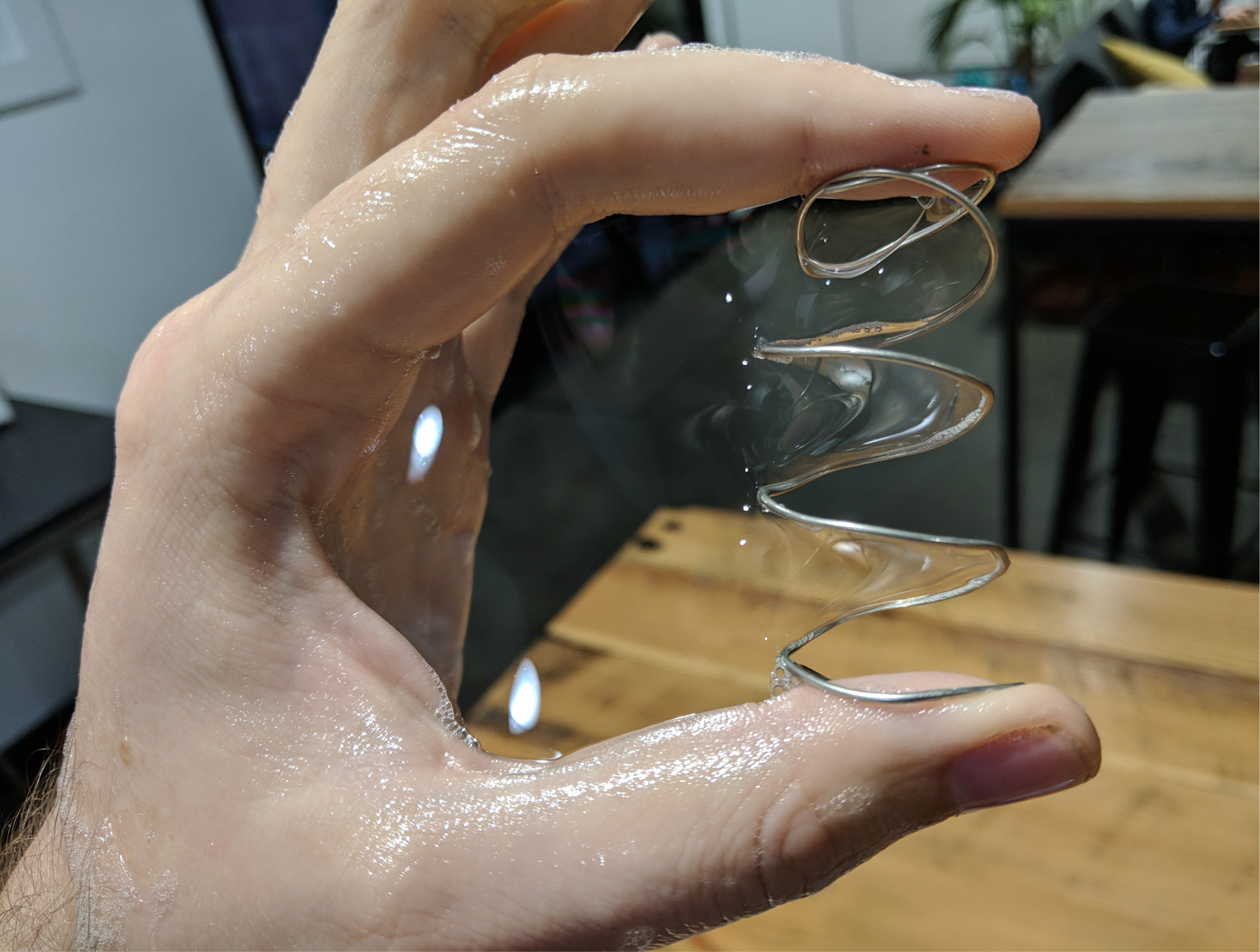To most, the computational power of bath water is essentially zero; but if you have a very unique problem to solve, it can be superior to a modern computer.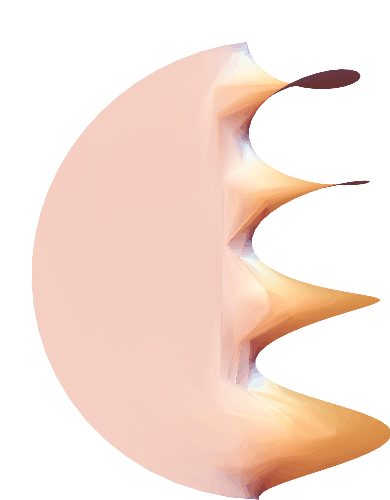# The Nature of Computation

When it comes down to it, the most obvious applications of quantum computers seem trivial—naturally, a computer built of photons will be effective at simulating photons. Quantum computers are no more a cure-all for general computation than soapy water is for black hole physics, but this apparent triviality clarifies our challenge.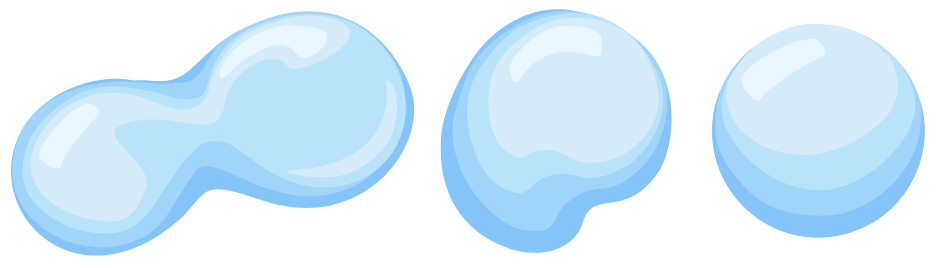The minimal surface problem can easily be mapped onto the surface energy of soap bubbles, which follows classical physics. We can piggyback nature to perform our calculation for us, by expressing it in the language of classical mechanics.

In a real sense, quantum computers do not compute at all, but simply carry on according to the laws of physics. The extent to which quantum computing can revolutionize computation depends on our finding ways to restate computational problems in equivalent quantum systems.

For some problems, like simulating the chemical bonds in a molecule, this is relatively straightforward. For other problems, like searching a database or factoring an integer, finding the bridge is much more subtle. This is why we'll spend much of the next chapter learning the relevant laws of quantum mechanics, while keeping close to the framework defined by classical computing.

# The Nature of Computation

×